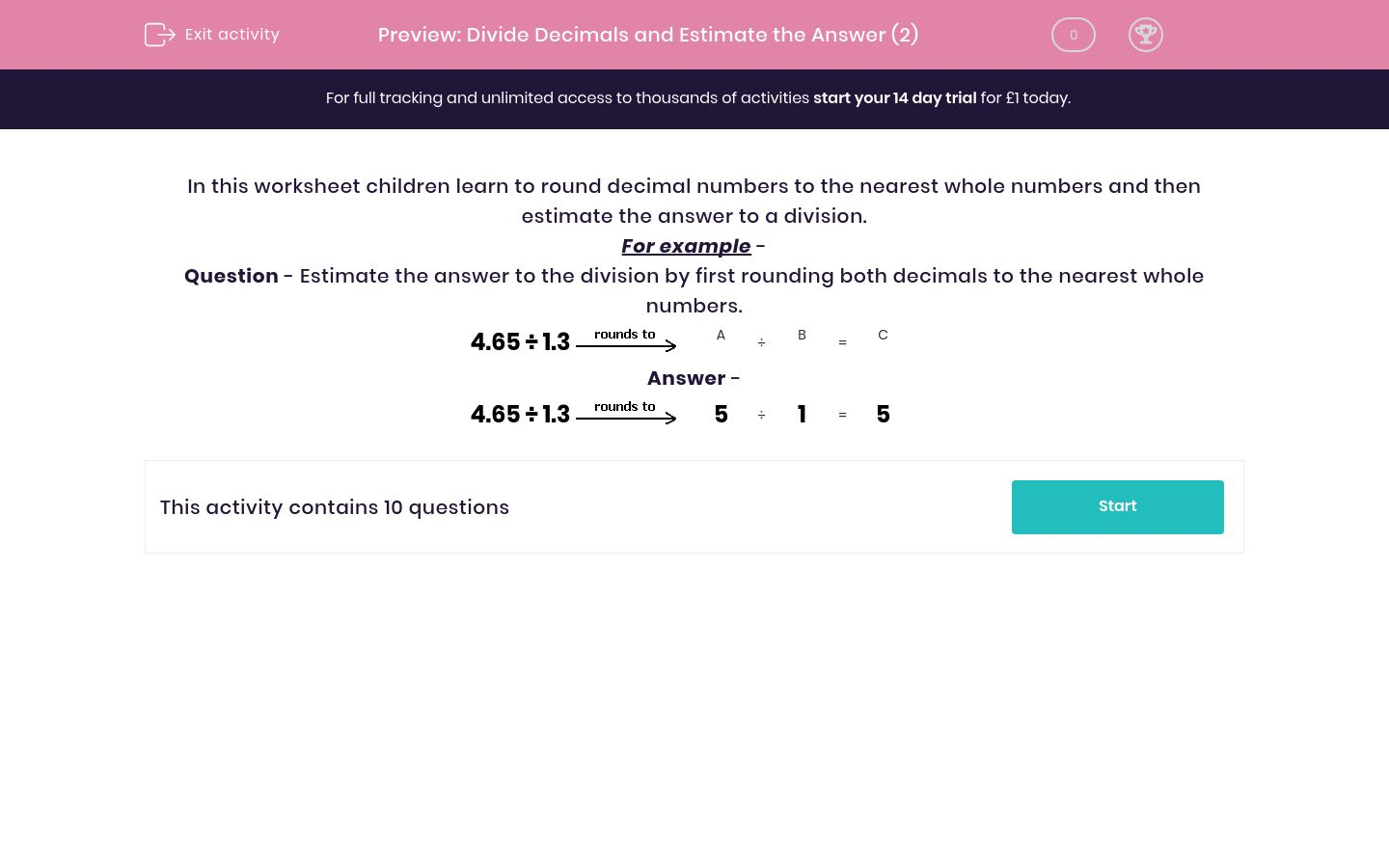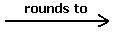# Divide Decimals and Estimate the Answer (2)

In this worksheet, students learn to round decimal numbers to the nearest whole numbers in order to estimate the answer to a division.Key stage:  KS 2

Curriculum topic:   Maths and Numerical Reasoning

Curriculum subtopic:   Decimals

Difficulty level:### QUESTION 1 of 10

In this worksheet children learn to round decimal numbers to the nearest whole numbers and then estimate the answer to a division.

For example -

Question - Estimate the answer to the division by first rounding both decimals to the nearest whole numbers.

4.65 ÷ 1.3A
÷
 B
=
 C

4.65 ÷ 1.35
÷
 1
=
 5

Estimate the answer to the division by first rounding both decimals to the nearest whole numbers.

7.53 ÷ 2.62A
÷
 B
=
 C

Remember to put your answers in the same sequence as the question!

For example, will be 7.53 to the nearest whole number and B will be 2.62...

## Column B

A
8
B
2.7
C
3

Estimate the answer to the division by first rounding both decimals to the nearest whole numbers.

1.96 ÷ 1.45A
÷
 B
=
 C

Remember to put your answers in the same sequence as the question!

For example, will be 1.96 to the nearest whole number and B will be 1.45...

## Column B

A
1
B
2
C
2

Estimate the answer to the division by first rounding both decimals to the nearest whole numbers.

3 ÷ 1.92A
÷
 B
=
 C

Remember to put your answers in the same sequence as the question!

For example, will be 3 to the nearest whole number and B will be 1.92...

## Column B

A
1.5
B
2
C
3

Estimate the answer to the division by first rounding both decimals to the nearest whole numbers.

5.88 ÷ 3.62A
÷
 B
=
 C

Remember to put your answers in the same sequence as the question!

## Column B

A
6
B
4
C
1.5

Estimate the answer to the division by first rounding both decimals to the nearest whole numbers.

2.27 ÷ 1.24A
÷
 B
=
 C

Remember to put your answers in the same sequence as the question!

## Column B

A
2
B
1
C
2

Estimate the answer to the division by first rounding both decimals to the nearest whole numbers.

11.7 ÷ 2.32A
÷
 B
=
 C

Remember to put your answers in the same sequence as the question!

## Column B

A
2
B
6
C
12

Estimate the answer to the division by first rounding both decimals to the nearest whole numbers.

2.32 ÷ 1.13A
÷
 B
=
 C

Remember to put your answers in the same sequence as the question!

## Column B

A
2
B
2
C
1

Estimate the answer to the division by first rounding both decimals to the nearest whole numbers.

6.7 ÷ 5.39A
÷
 B
=
 C

Remember to put your answers in the same sequence as the question!

## Column B

A
1.4
B
5
C
7

Estimate the answer to the division by first rounding both decimals to the nearest whole numbers.

4.69 ÷ 1.68A
÷
 B
=
 C

Remember to put your answers in the same sequence as the question!

## Column B

A
2
B
2.5
C
5

Estimate the answer to the division by first rounding both decimals to the nearest whole numbers.

1.83 ÷ 1.54A
÷
 B
=
 C

Remember to put your answers in the same sequence as the question!

## Column B

A
2
B
1
C
2
• Question 1

Estimate the answer to the division by first rounding both decimals to the nearest whole numbers.

7.53 ÷ 2.62A
÷
 B
=
 C

Remember to put your answers in the same sequence as the question!

For example, will be 7.53 to the nearest whole number and B will be 2.62...

## Column B

A
8
B
3
C
2.7
• Question 2

Estimate the answer to the division by first rounding both decimals to the nearest whole numbers.

1.96 ÷ 1.45A
÷
 B
=
 C

Remember to put your answers in the same sequence as the question!

For example, will be 1.96 to the nearest whole number and B will be 1.45...

## Column B

A
2
B
1
C
2
• Question 3

Estimate the answer to the division by first rounding both decimals to the nearest whole numbers.

3 ÷ 1.92A
÷
 B
=
 C

Remember to put your answers in the same sequence as the question!

For example, will be 3 to the nearest whole number and B will be 1.92...

## Column B

A
3
B
2
C
1.5
• Question 4

Estimate the answer to the division by first rounding both decimals to the nearest whole numbers.

5.88 ÷ 3.62A
÷
 B
=
 C

Remember to put your answers in the same sequence as the question!

## Column B

A
6
B
4
C
1.5
• Question 5

Estimate the answer to the division by first rounding both decimals to the nearest whole numbers.

2.27 ÷ 1.24A
÷
 B
=
 C

Remember to put your answers in the same sequence as the question!

## Column B

A
2
B
1
C
2
• Question 6

Estimate the answer to the division by first rounding both decimals to the nearest whole numbers.

11.7 ÷ 2.32A
÷
 B
=
 C

Remember to put your answers in the same sequence as the question!

## Column B

A
12
B
2
C
6
• Question 7

Estimate the answer to the division by first rounding both decimals to the nearest whole numbers.

2.32 ÷ 1.13A
÷
 B
=
 C

Remember to put your answers in the same sequence as the question!

## Column B

A
2
B
1
C
2
• Question 8

Estimate the answer to the division by first rounding both decimals to the nearest whole numbers.

6.7 ÷ 5.39A
÷
 B
=
 C

Remember to put your answers in the same sequence as the question!

## Column B

A
7
B
5
C
1.4
• Question 9

Estimate the answer to the division by first rounding both decimals to the nearest whole numbers.

4.69 ÷ 1.68A
÷
 B
=
 C

Remember to put your answers in the same sequence as the question!

## Column B

A
5
B
2
C
2.5
• Question 10

Estimate the answer to the division by first rounding both decimals to the nearest whole numbers.

1.83 ÷ 1.54A
÷
 B
=
 C

Remember to put your answers in the same sequence as the question!

## Column B

A
2
B
2
C
1
---- OR ----

Sign up for a £1 trial so you can track and measure your child's progress on this activity.

### What is EdPlace?

We're your National Curriculum aligned online education content provider helping each child succeed in English, maths and science from year 1 to GCSE. With an EdPlace account you’ll be able to track and measure progress, helping each child achieve their best. We build confidence and attainment by personalising each child’s learning at a level that suits them.

Get started